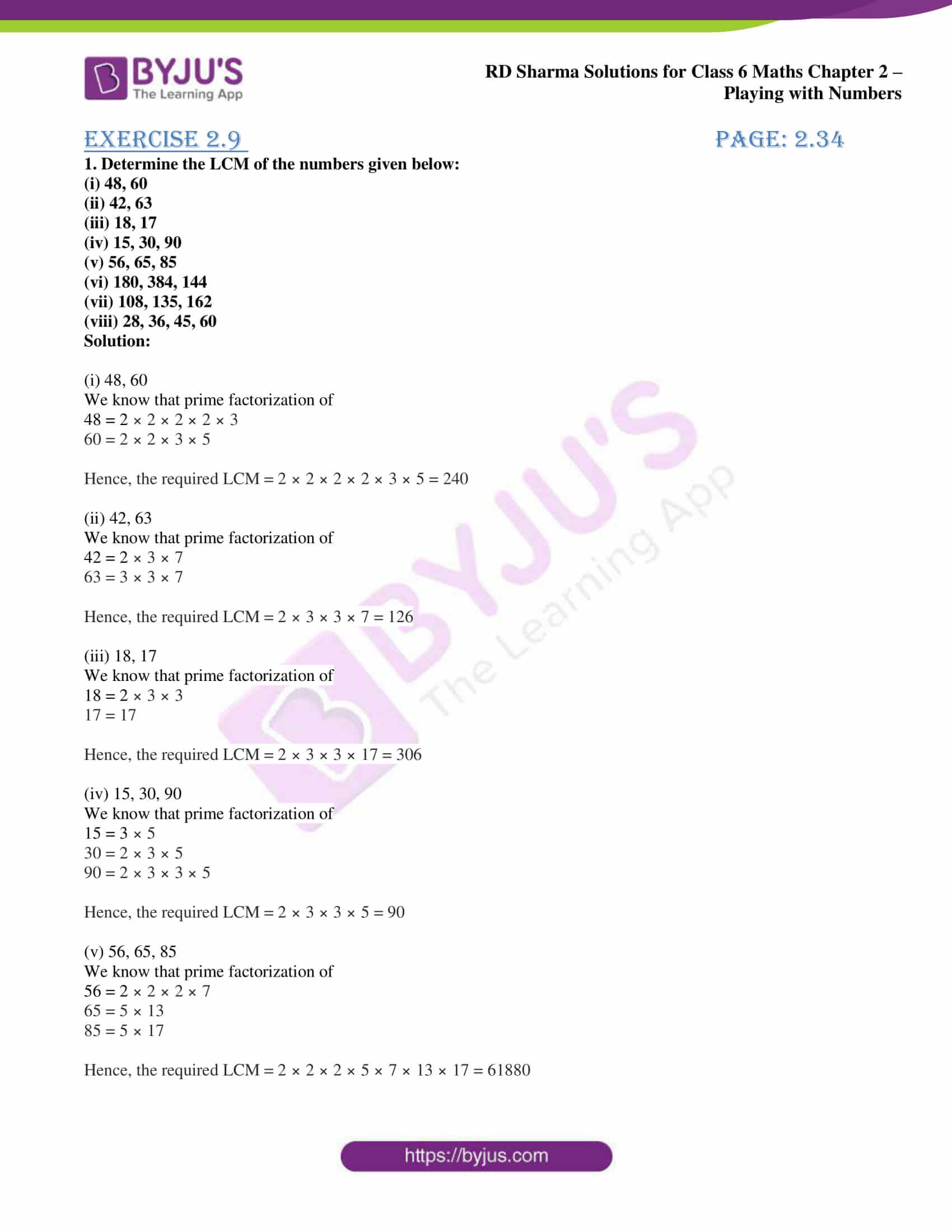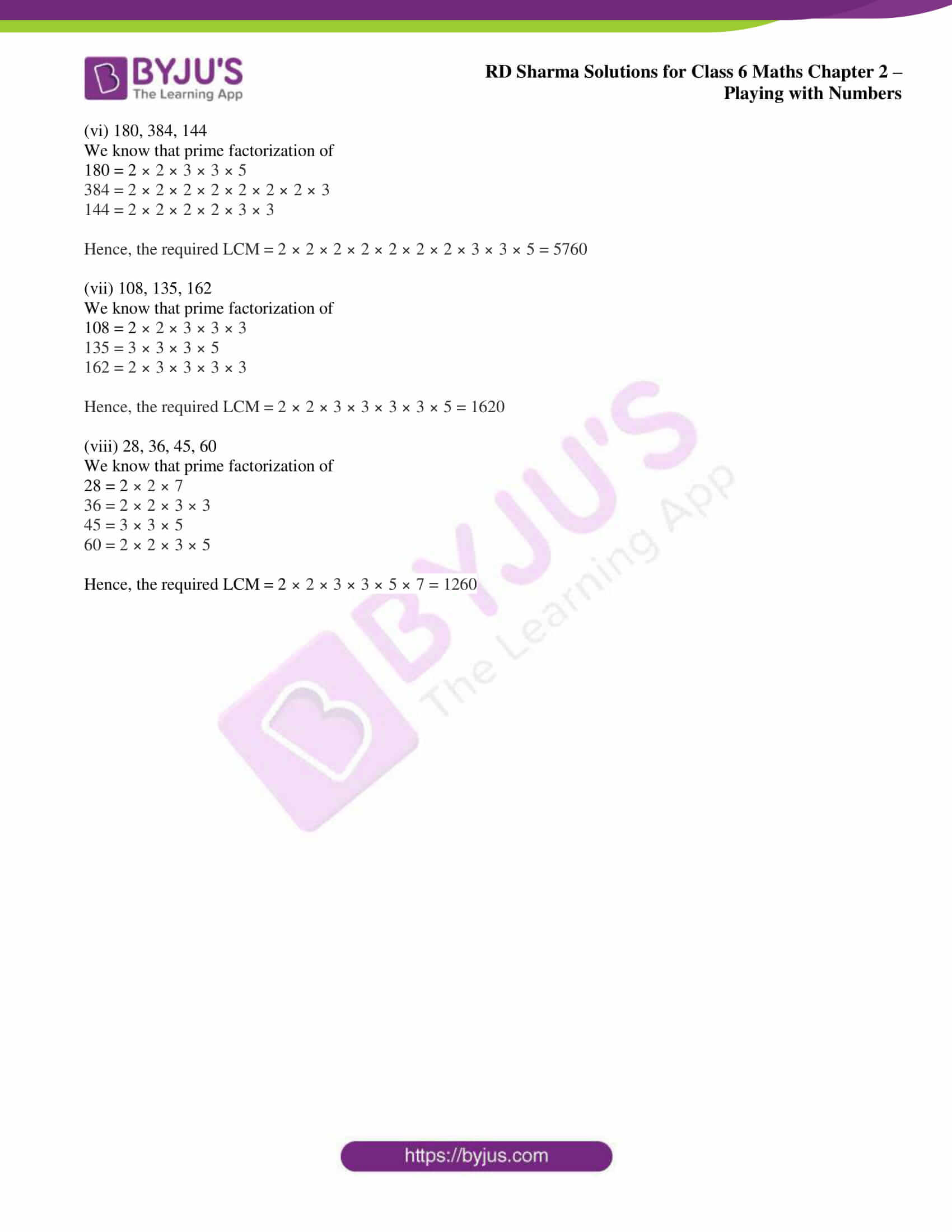# RD Sharma Solutions for Class 6 Maths Chapter 2: Playing with Numbers Exercise 2.9

## RD Sharma Solutions for Class 6 Maths Exercise 2.9 PDF

The solutions are designed by the subject matter experts at BYJU’S based on the current CBSE guidelines. The students are provided with exercise wise solutions to help them gain better knowledge about the concepts covered. Practising the examples and problems mainly improves efficiency while solving among students. PDF of solutions can be downloaded for free and used while solving problems of RD Sharma textbook. Self study method is improved among students which help in performing better in the exam. RD Sharma Solutions for Class 6 Maths Chapter 2 Playing with Numbers Exercise 2.9 are provided here.

## RD Sharma Solutions for Class 6 Maths Chapter 2: Playing with Numbers Exercise 2.9 Download PDF## Access answers to Maths RD Sharma Solutions for Class 6 Chapter 2: Playing with Numbers Exercise 2.9

1. Determine the LCM of the numbers given below:

(i) 48, 60

(ii) 42, 63

(iii) 18, 17

(iv) 15, 30, 90

(v) 56, 65, 85

(vi) 180, 384, 144

(vii) 108, 135, 162

(viii) 28, 36, 45, 60

Solution:

(i) 48, 60

We know that prime factorization of

48 = 2 × 2 × 2 × 2 × 3

60 = 2 × 2 × 3 × 5

Hence, the required LCM = 2 × 2 × 2 × 2 × 3 × 5 = 240

(ii) 42, 63

We know that prime factorization of

42 = 2 × 3 × 7

63 = 3 × 3 × 7

Hence, the required LCM = 2 × 3 × 3 × 7 = 126

(iii) 18, 17

We know that prime factorization of

18 = 2 × 3 × 3

17 = 17

Hence, the required LCM = 2 × 3 × 3 × 17 = 306

(iv) 15, 30, 90

We know that prime factorization of

15 = 3 × 5

30 = 2 × 3 × 5

90 = 2 × 3 × 3 × 5

Hence, the required LCM = 2 × 3 × 3 × 5 = 90

(v) 56, 65, 85

We know that prime factorization of

56 = 2 × 2 × 2 × 7

65 = 5 × 13

85 = 5 × 17

Hence, the required LCM = 2 × 2 × 2 × 5 × 7 × 13 × 17 = 61880

(vi) 180, 384, 144

We know that prime factorization of

180 = 2 × 2 × 3 × 3 × 5

384 = 2 × 2 × 2 × 2 × 2 × 2 × 2 × 3

144 = 2 × 2 × 2 × 2 × 3 × 3

Hence, the required LCM = 2 × 2 × 2 × 2 × 2 × 2 × 2 × 3 × 3 × 5 = 5760

(vii) 108, 135, 162

We know that prime factorization of

108 = 2 × 2 × 3 × 3 × 3

135 = 3 × 3 × 3 × 5

162 = 2 × 3 × 3 × 3 × 3

Hence, the required LCM = 2 × 2 × 3 × 3 × 3 × 3 × 5 = 1620

(viii) 28, 36, 45, 60

We know that prime factorization of

28 = 2 × 2 × 7

36 = 2 × 2 × 3 × 3

45 = 3 × 3 × 5

60 = 2 × 2 × 3 × 5

Hence, the required LCM = 2 × 2 × 3 × 3 × 5 × 7 = 1260

### RD Sharma Solutions for Class 6 Maths Chapter 2 – Playing with Numbers Exercise 2.9

RD Sharma Solutions Class 6 Maths Chapter 2 Playing with Numbers Exercise 2.9 help students to understand the methods used in finding LCM by prime factorization and common division method.

### Key features of RD Sharma Solutions for Class 6 Maths Chapter 2: Playing with Numbers Exercise 2.9

• The solutions mainly help students understand the variety of problems that would arise using a single concept.
• The examples before each exercise improves accuracy and efficiency in solving exercise wise problems.
• RD Sharma Solutions created by subject experts help students understand the concepts in a better way.
• Time management and self study methods are improved which are important from the exam point of view.